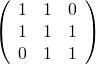## Solving the nearest correlation matrix problem using Python

November 17th, 2014 | Categories: general math, Linear Algebra, NAG Library, Numerics, programming, python | Tags:

Given a symmetric matrix such asWhat’s the nearest correlation matrix? A 2002 paper by Manchester University’s Nick Higham which answered this question has turned out to be rather popular! At the time of writing, Google tells me that it’s been cited 394 times.

Last year, Nick wrote a blog post about the algorithm he used and included some MATLAB code. He also included links to applications of this algorithm and implementations of various NCM algorithms in languages such as MATLAB, R and SAS as well as details of the superb commercial implementation by The Numerical algorithms group.

I noticed that there was no Python implementation of Nick’s code so I ported it myself.

Here’s an example IPython session using the module

In : from nearest_correlation import nearcorr

In : import numpy as np

In : A = np.array([[2, -1, 0, 0],
...:               [-1, 2, -1, 0],
...:               [0, -1, 2, -1],
...:               [0, 0, -1, 2]])

In : X = nearcorr(A)

In : X
Out:
array([[ 1.        , -0.8084125 ,  0.1915875 ,  0.10677505],
[-0.8084125 ,  1.        , -0.65623269,  0.1915875 ],
[ 0.1915875 , -0.65623269,  1.        , -0.8084125 ],
[ 0.10677505,  0.1915875 , -0.8084125 ,  1.        ]])

This module is in the early stages and there is a lot of work to be done. For example, I’d like to include a lot more examples in the test suite, add support for the commercial routines from NAG and implement other algorithms such as the one by Qi and Sun among other things.

Hopefully, however, it is just good enough to be useful to someone. Help yourself and let me know if there are any problems. Thanks to Vedran Sego for many useful comments and suggestions.